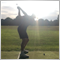# Why is this not returning true ?39

```bool calcLong3;
bool calcShort3;

bool calcLong3()
{
double RSI = iRSI(_Symbol,_Period,14,PRICE_CLOSE,0);
if (RSI<70)
return true;
else
return false;
}

bool calcShort3()
{
double RSI = iRSI(_Symbol,_Period,14,PRICE_CLOSE,0);
if (RSI>30)
return true;
else
return false;
}

void OnTick()
{
if(calcLong3 == true)
{
}
}

```

no matter what the RSI is it always returns false, where have i gone wrong ??Moderator
30417

Please edit your post and use the code button (Alt+S) when pasting code.
EDIT your original post, please do not just post the code correctly in a new post.Moderator
30417

It is a bad idea to name functions the same as other variables as it leads to errors such as this.

```   if(calcLong3 == true)
{
}```

You do not assign any value to calcLong339

Keith Watford:

It is a bad idea to name functions the same as other variables as it leads to errors such as this.

You do not assign any value to calcLong3

Hi Keith, thanks for the fast reply, i am extremely new to coding and learning as i go, i wanted calcLong3 to return true does it need a numerical value instead then, like 1 = true and 0 = false ?Moderator
30417

George Johnson:
Hi Keith, thanks for the fast reply, i am extremely new to coding and learning as i go, i wanted calcLong3 to return true does it need a numerical value instead then, like 1 = true and 0 = false ?

No, never use an int when it should be a bool, that is another bad practice.

Keith Watford:

It is a bad idea to name functions the same as other variables as it leads to errors such as this.

You do not assign any value to calcLong3

`bool calcLong3;`

This ^ is a variable

```bool calcLong3()
{
double RSI = iRSI(_Symbol,_Period,14,PRICE_CLOSE,0);
if (RSI<70)
return true;
else
return false;
}```

This ^ is a function

```   if(calcLong3 == true)
{
}```

You have not assigned any value to calcLong3

You have not used the function at all.

```   if(calcLong3() == true)
{
}```

will call the function and use the value.24798

1.  Your code ```      if (RSI<70)       return true;       else       return false;``` simplified `      return RSI<70;`
2. `   if(calcLong3() == true)`

You should be able to read your code out loud and have it make sense. You would never write if( (2+2 == 4) == true) would you? if(2+2 == 4) is sufficient. So don't write if(bool == true), just use if(bool) or if(!bool). Code becomes self documenting when you use meaningful variable names, like bool isLongEnabled where as Long_Entry sounds like a trigger price or a ticket number and “if long entry” is an incomplete sentence.Moderator
30417

Please do not open a new topic when it is basically the same code as in this topic.
I will delete your new topic and move it here.Moderator
30417

George Johnson:

How to make multiple conditions trigger a buy

```bool calcLong1;
bool calcLong2;
bool calcLong3;
bool calcLong4;
bool calcLong5;
bool calcLong6;

bool calcLong3()
{
double RSI = iRSI(_Symbol,_Period,14,PRICE_CLOSE,0);
return RSI<70;
}

void OnTick()

{
if((calcLong1()||calcLong2() == true) && (calcLong3()==true) && (calcLong4()==true) && (calcLong5() == true))
{
if(CountPositions()<1)
{
Print ("LONG");
}
}
}

int CountPositions()
{
int NumberOfPositions=0;

for(int i=OrdersTotal()-1; i>= 0; i--)
{

string CurrencyPair=OrderSymbol();

if(_Symbol==CurrencyPair)

{
NumberOfPositions=NumberOfPositions+1;
}
}
return NumberOfPositions;
};
```
say there was multiple functions that returned true and false values for calclong1 and calclong2 and calclong3 ... etc, how would i code it so that the print long would only trigger if either calclong1 was true or calclong2 was true and the rest have to also all be true up to calclong5, and if coint positions is less than 1, im very new to coding so im sure ive made lots of mistakes but thanks for any help.

Copied aboveModerator
30417

I have already told you that it is a bad idea to name variables the same as functions as it can often lead to mistakes.

Yet you are still doing it.

Why do you even need these variables?

```bool calcLong1;
bool calcLong2;
bool calcLong3;
bool calcLong4;
bool calcLong5;
bool calcLong6;```

You don't seem to use them in the code.Moderator
30417

`if(OrderType()==OP_BUY||OP_SELL)`

You cannot do this.

The correct way is

`if(OrderType()==OP_BUY || OrderType()==OP_SELL)`Moderator
30417

George Johnson:
....how would i code it......  if either calclong1 was true or calclong2 was true and the rest have to also all be true up to calclong5.....

You would code it exactly as you would say it

if  calclong1==true Or  calclong2==true And  calclong3 ==true  And  calclong4 ==true  And  calclong5 ==true

`if ( calclong1==true || calclong2==true && calclong3 ==true  && calclong4 ==true  && calclong5 ==true)`

now that is a bit ambiguous as it often can be when mixing || and &&

so we put in some extra brackets to make it clear

`if ( (calclong1==true || calclong2==true) && calclong3 ==true  && calclong4 ==true  && calclong5 ==true)`

As William has pointed out with bools or boolean results the ==true is not really necessary

`if ( (calclong1 || calclong2) && calclong3  && calclong4  && calclong5)`